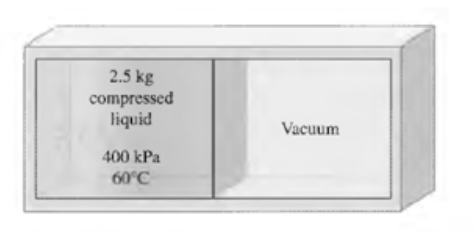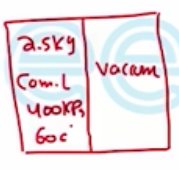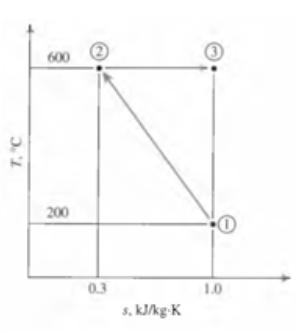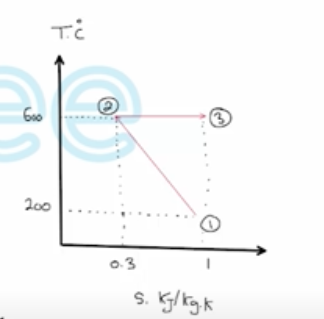Need Help?

Subscribe to Thermodynamics

###### \${selected_topic_name}
• Notes
• Comments & Questions

$\begin{array}{l}{\text { A rigid tank is divided into two equal parts by a }} \\ {\mathrm{v}\langle\mathrm{c}>>\text { partition. One part of the tank contains } 2.5 \mathrm{kg} \text { of }} \\ {\text { compressed liquid water at } 400 \mathrm{kPa} \text { and } 60^{\circ} \mathrm{C} \text { while the other }} \\ {\text { part is evacuated. The partition is now removed, and the }} \\ {\text { water expands to fill the entire tank. Determine the entropy }} \\ {\text { change of water during this process, if the final pressure in }} \\ {\text { the tank is } 40 \mathrm{kPa} \text { . }}\end{array}$$\left.\begin{array}{l}{P_{1}=400 \mathrm{kPa}} \\ {T_1=60 \mathrm{C}^{\circ}}\end{array}\right] \rightarrow \mathrm{A}-4$

$V_{1}=V_{f @ 60 c^{\circ}}=0.001017 m^3/kg$

$S_{1}=S_{f @ 60 c^{\circ}}=0.8313kJ/kg.k$

$V_{2}=2 V_{1}=2 * 0.001017=0.002034 \mathrm{m}^{3} \mathrm{/kg}$

$\left.\begin{array}{l}{P_{2}=40 \mathrm{kPa}} \\ {\mathrm{V}_{2}=0.002034}\end{array}\right]\rightarrow X_{2}=\frac{V_{2}-V_{f}}{V_{f y}}=\frac{0.002034-0.0026}{3.993-0.001026}=0.0002524$

$S_{2}=S_{f}+X_{2} S_{f{y}}$

$=1.0261+(0.0002524)(6.6430)=1.0278 \mathrm{kJ} / \mathrm{kg.k}$

$\Delta S=m\left(s_{2}-s_{1}\right)=(2.5)(1.0278-0.8313)=$

$∴ \Delta S=0.492 \mathrm{KJ} / \mathrm{K}$

$\begin{array}{l}{\text { Refrigerant- } 134 a \text { is expanded isentropically from } 800 \mathrm{kPa} \text { and } 60^{\circ} \mathrm{C} \text { at }} \\ {\text { the inlet of a steady-flow turbine to } 100 \mathrm{kPa} \text { at the outlet. The outlet area is } 1 \mathrm{m} 2,}\end{array}$

$\begin{array}{l}{\text { and the inlet area is } 0.5 \mathrm{m} 2 \text { . Calculate the inlet and outlet velocities when the mass }} \\ {\text { flow rate is } 0.5 \mathrm{kg} / \mathrm{s} \text { . }}\end{array}$

$V=\frac{\dot m v}{A}$

(1) $\left.\begin{array}{l}{P_{1}=800 \mathrm{kPa}} \\ {T_{1}=60 c^{\circ}}\end{array}\right\}\rightarrow \quad A-13 \rightarrow$$\begin{array}{l}{v_{1}=0.02997 \mathrm{m}^{3} / \mathrm{kg}} \\ {s_{1}=1.0110 \mathrm{k} \mathrm{J} / \mathrm{kg} . \mathrm{K}}\end{array}$

(2) $\left.\begin{array}{l}{P_{1}=100 \mathrm{kPa}} \\ {S_{2}=S_1=1.0110 kJ/kg.k}\end{array}\right\}\rightarrow \quad A-13 \rightarrow v_2=0.2098 m^3/kg$

$V_{1}=\frac{\dot{m}_{1} v_{1}}{A_{1}}=\frac{0.5(0.02947)}{0.5}=0.03 m/s$

$V_{2}=\frac{\dot{m}_{2} v_{2}}{A_{2}}=\frac{0.5 * 0.2078}{1}=0.105 \mathrm{m} / \mathrm{s}$

$\begin{array}{l}{\text { Calculate the heat transfer, in } \mathrm{k} \mathrm{J} / \mathrm{kg} \text { , for the reversible steady-flow process }} \\ {13-\text { shown in Fig. } \mathrm{P} 749 .}\end{array}$$q ? ?$

$q=\int_{1}^{2} T d s+\int_{2}^{3} T d s$

$=\frac{T_{1}+T_{2}}{2}\left(S_{2}-S_{1}\right)+T_{2}\left(S_{3}-S_{2}\right)$

$=\frac{(200+273)+(600+273)}{2}(0.3-1)$

$+600(1-0.3)=140 \quad \mathrm{kJ} / \mathrm{kg}$

No comments yet